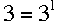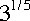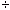SEARCH HOMEMath Central Quandaries & QueriesQuestion from Annie, a student: How do I transform the equation 3 divided by 3 to it's fifth root to simple radical form (getting the radical out of the denominator)?Hi Annie,

The numerator isand the denominator is.

Now you need to use one of the laws of exponents. They are

abac = ab + c
abac = ab - c as long as a ≠ 0, and
(ab)c = abc

PennyMath Central is supported by the University of Regina and The Pacific Institute for the Mathematical Sciences.